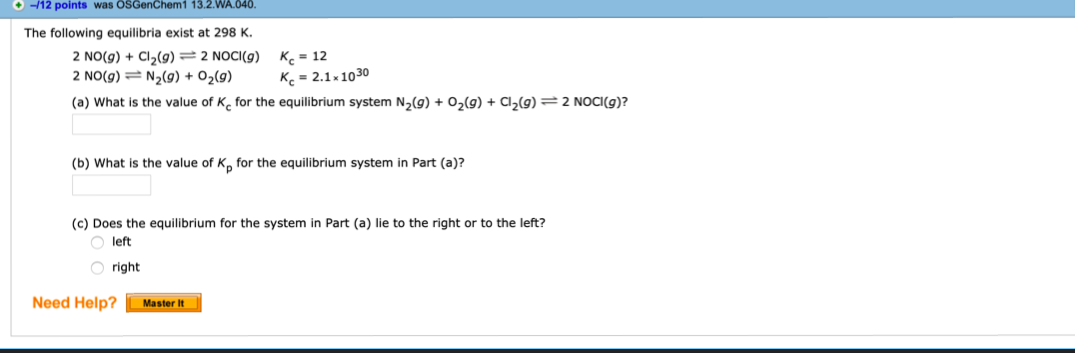# The following equilibria exist at 298 K. 2 NO(g) + Cl2(g) - 2 NOCI(g) Kc = 12 2 NO(g) = N2(g) + 02(g) Kc = 2.1 x 1030 (a) What is the value of Kc for the equilibrium system N2(g) + 02(g) + Cl2(g) -= 2 NOCI(g)? (b) What is the value of K for the equilibrium system in Part (a)? (c) Does the equilibrium for the system in Part (a) lie to the right or to the left? left right